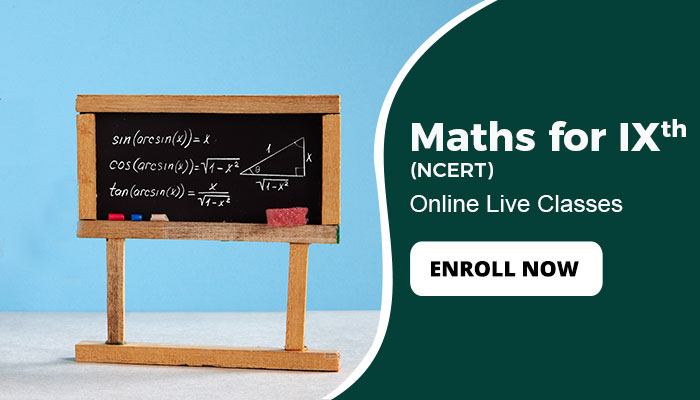# NCERT Maths class 9

CategoriesSYLLABUS

1. NUMBER SYSTEMS

1.1 Introduction

1.2 Irrational Numbers

1.3 Real Numbers and their Decimal Expansions

1.4 Representing Real Numbers on the Number Line

1.5 Operations on Real Numbers

1.6 Laws of Exponents for Real Numbers

1.7 Summary

2. POLYNOMIALS

2.1 Introduction

2.2 Polynomials in One Variable

2.3 Zeroes of a Polynomial

2.4 Remainder Theorem

2.5 Factorisation of Polynomials

2.6 Algebraic Identities

2.7 Summary

3. COORDINATE GEOMETRY

3.1 Introduction

3.2 Cartesian System

3.3 Plotting a Point in the Plane if its Coordinates are given

3.4 Summary

4. LINEAR EQUATIONS IN TWO VARIABLES

4.1 Introduction

4.2 Linear Equations

4.3 Solution of a Linear Equation

4.4 Graph of a Linear Equation in Two Variables

4.5 Equations of Lines Parallel to x-axis and y-axis

4.6 Summary

5. INTRODUCTION TO EUCLID’S GEOMETRY

5.1 Introduction

5.2 Euclid’s Definitions, Axioms and Postulates

5.3 Equivalent Versions of Euclid’s Fifth Postulate

5.4 Summary

6. LINES AND ANGLES

6.1 Introduction

6.2 Basic Terms and Definitions

6.3 Intersecting Lines and Non-intersecting Lines

6.4 Pairs of Angles

6.5 Parallel Lines and a Transversal

6.6 Lines Parallel to the same Line

6.7 Angle Sum Property of a Triangle

6.8 Summary

7. TRIANGLES

7.1 Introduction

7.2 Congruence of Triangles

7.3 Criteria for Congruence of Triangles

7.4 Some Properties of a Triangle

7.5 Some More Criteria for Congruence of Triangles

7.6 Inequalities in a Triangle

7.7 Summary

8.1 Introduction

8.2 Angle Sum Property of a Quadrilateral

8.4 Properties of a Parallelogram

8.5 Another Condition for a Quadrilateral to be a Parallelogram

8.6 The Mid-point Theorem

8.7 Summary

9. AREAS OF PARALLELOGRAMS AND TRIANGLES

9.1 Introduction

9.2 Figures on the same Base and Between the same Parallels

9.3 Parallelograms on the same Base and between the same Parallels

9.4 Triangles on the same Base and between the same Parallels

9.5 Summary

10. CIRCLES

10.1 Introduction

10.2 Circles and its Related Terms : A Review

10.3 Angle Subtended by a Chord at a Point

10.4 Perpendicular from the Centre to a Chord

10.5 Circle through Three Points

10.6 Equal Chords and their Distances from the Centre

10.7 Angle Subtended by an Arc of a Circle

10.9 Summary

11. CONSTRUCTIONS

11.1 Introduction

11.2 Basic Constructions

11.3 Some Constructions of Triangles

11.4 Summary

12. HERON’S FORMULA

12.1 Introduction

12.2 Area of a Triangle – by Heron’s Formula 199

12.3 Application of Heron’s Formula in finding Areas of Quadrilaterals

12.4 Summary

13. SURFACE AREAS AND VOLUMES

13.1 Introduction

13.2 Surface Area of a Cuboid and a Cube

13.3 Surface Area of a Right Circular Cylinder

13.4 Surface Area of a Right Circular Cone

13.5 Surface Area of a Sphere

13.6 Volume of a Cuboid

13.7 Volume of a Cylinder

13.8 Volume of a Right Circular Cone

13.9 Volume of a Sphere

14. STATISTICS

14.1 Introduction

14.2 Collection of Data

14.3 Presentation of Data

14.4 Ggraphical Representation of Data

14.5 Measures of Central Tendency

14.6 Summary

15. PROBABILITY

15.1 Introduction

15.2 Probability – an Experimental Approach

15.3 Summary

APPENDIX – 1 PROOFS IN MATHEMATICS

A1.1 Introduction

A1.2 Mathematically Acceptable Statements

A1.3 Deductive Reasoning

A1.4 Theorems, Conjectures and Axioms

A1.5 What is a Mathematical Proof?

A1.6 Summary

APPENDIX – 2 INTRODUCTION TO MATHEMATICAL MODELLING

A2.1 Introduction

A2.2 Review of Word Problems

A2.3 Some Mathematical Models

A2.4 The Process of Modelling, its Advantages and Limitations

A2.5 Summary